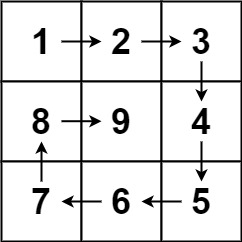# ARTS 打卡第 5 周

• 2023-09-17
浙江
• 本文字数：1107 字

阅读完需：约 4 分钟# 1.Algorithm: 每周至少做一个 LeetCode 的算法题

## 题目 ：螺旋矩阵 II## 解法总结

vector<vector<int>> generateMatrix(int n) {        vector<vector<int>> res(n, vector<int>(n,0));         int l = 0, r = n - 1, t = 0, b = n - 1;         int num = 1;         int tar = n * n;         int i, j;         while (num <= tar) {             // 下面开始的四个for就是模拟转了一圈             // 模拟填充上行从左到右(左闭右闭)             for (j = l; j <= r; j++) {                 res[t][j] = num++;             }             t++;             // 模拟填充右列从上到下(左闭右闭)             for (i = t; i <= b; i++) {                 res[i][r] = num++;             }             r--;             // 模拟填充下行从右到左(左闭右闭)             for (j = r; j >= l; j--) {                 res[b][j] = num++;             }             b--;             // 模拟填充左列从下到上(左闭右闭)             for (i = b; i >= t; i--) {                 res[i][l] = num++;             }             l++;         }     return res;     } 

1. Review: 阅读并点评至少一篇英文技术文章

# 4.Share: 分享一篇有观点和思考的技术文章/观点## 评论